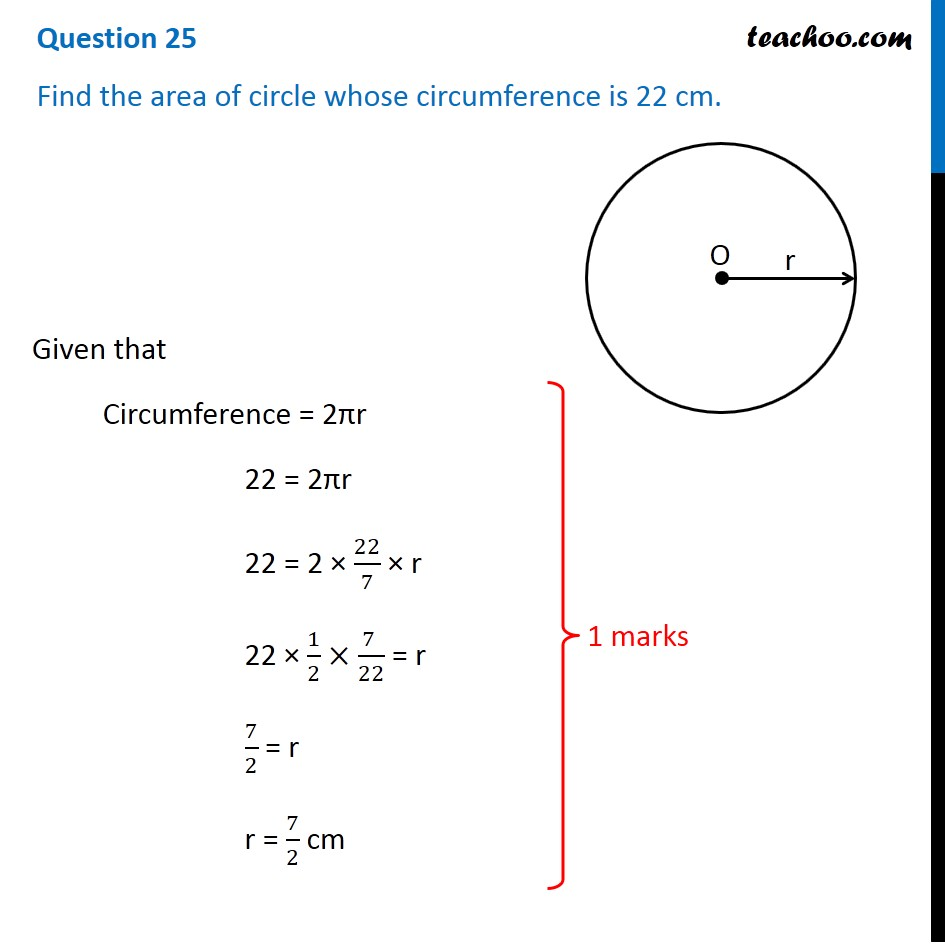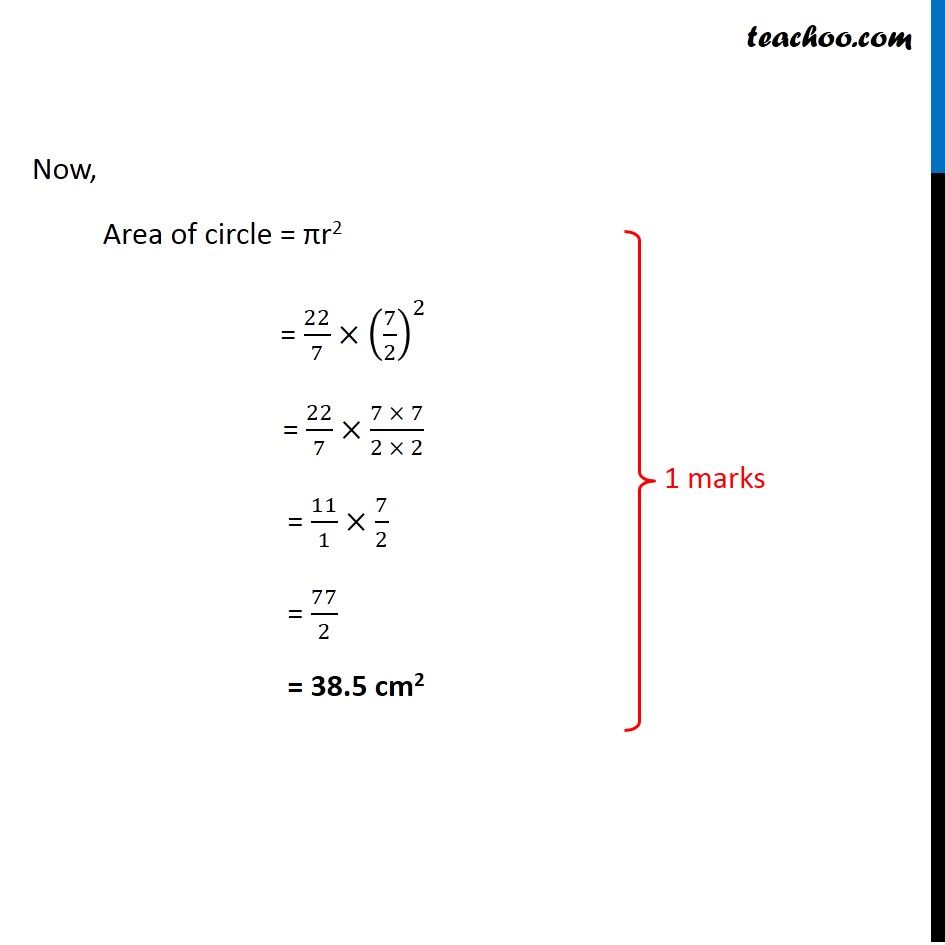CBSE Class 10 Sample Paper for 2020 Boards - Maths Basic

Class 10
Solutions of Sample Papers for Class 10 Boards

## Find the area of circle whose circumference is 22 cm.Note : This is similar to Ex 12.2, 2 of NCERT – Chapter 12 Class 10

Get live Maths 1-on-1 Classs - Class 6 to 12

### Transcript

Question 25 Find the area of circle whose circumference is 22 cm. Given that Circumference = 2πr 22 = 2πr 22 = 2 × 22/7 × r 22 × 1/2×(7 )/22 = r 7/2 = r r = 7/2 cm Now, Area of circle = πr2 = 22/7×(7/2)^2 = 22/7×(7 × 7)/(2 × 2) = 11/1×7/2 = 77/2 = 38.5 cm2